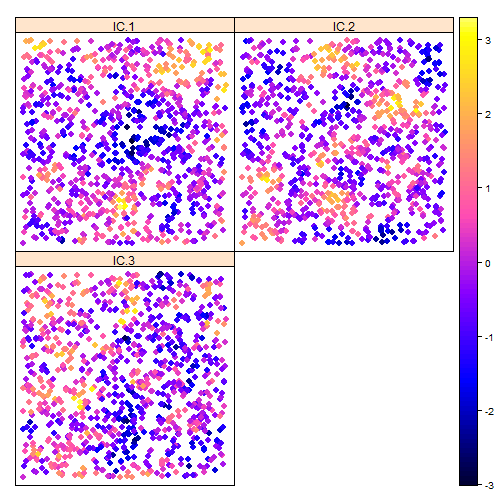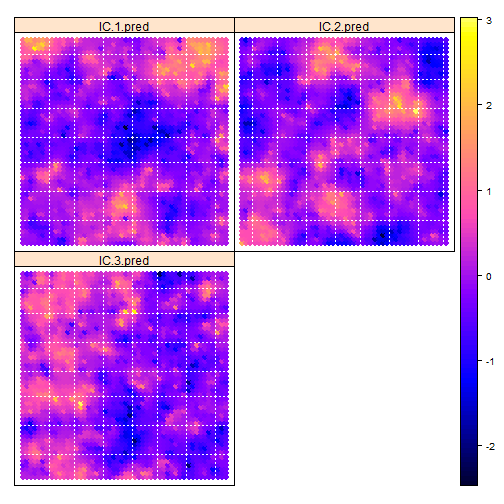# Spatial Blind Source Separation Framework

Blind Source Separation (BSS) is a well known multivariate data analysis tool. In BSS it is assumed that the observations are formed by a linear mixture of latent variables that are unobserved but show a clearer structure. The aim of BSS is to estimate those unobserved variables which in turn can be used for further analysis. This manual aims to firstly recap BSS for spatial data and then secondly introduce the R package SpatialBSS where Spatial Blind Source Separation is implemented.

## Spatial Blind Source Separation Model

In the Spatial Blind Source Separation (SBSS) model it is assumed that a $$p$$-variate random field $$X(s) = \{ X_1(s),\dots,X_p(s) \}$$ is a linear mixture of an unobserved random field $$Z(s) = \{ Z_1(s),\dots,Z_p(s) \}$$. Here $$s$$ denotes spatial locations in a domain $$s \in S \subseteq \mathbb{R}^d$$, where $$d$$ is the dimension of the domain. Usually $$d$$ equals two, which would for example correspond to x and y coordinates or longitude and latitude values. The following presented method is mainly designed to be used with irregular spatial data. Formally, the SBSS model is given by

$X(s) = \Omega Z(s) + \mu,$ where $$\Omega$$ is the so called mixing matrix and $$\mu$$ is some constant shift. The latent field $$Z(s)$$ shows a clearer structure than the original field $$X(s)$$. In particular, $$Z(s)$$ is assumed to have zero mean and unit covariance and the components of $$Z(s)$$ show no spatial cross-dependence. Meaning that $$\text{E}\{ Z(s) \} = 0$$, $$\text{Cov} \{ Z(s) \} = \text{E} \{ Z(s) Z(s)^\top \} = I_p$$ for all $$s \in \mathcal{S}$$ and $$\text{Cov} \{ Z(s_1), Z(s_2) \} = \text{E} \{ Z(s_1) Z(s_2)^\top \} = D(\lVert s_1 - s_2 \rVert)$$ for all $$s_1, s_2 \in \mathcal{S}$$ where $$D$$ is some diagonal matrix. Hence, $$Z(s)$$ is also a weakly-stationary random field.

Recovering the source random field $$Z(s)$$ is particularly beneficial in spatial prediction tasks such as various kriging methods. As the latent field shows no cross-dependence, univariate kriging can be applied on each component of $$Z(s)$$ individually. This approach avoids the complex modeling of cross-covariances and the use of CoKriging. In order to recover an estimate of the unmixing matrix $$W = \Omega ^ {-1}$$ local covariance matrices are used.

## Local Covariance Matrices and Spatial Kernel Functions

The aim of local covariance matrices is to quantify spatial dependence. This is achieved by the following definition:

$M(f) = \frac{1}{n} \sum_{i=1}^n \sum_{j=1}^n f(s_i - s_j) X(s_i) X(s_j)^\top$

where the sums range over all points in the domain and the locality is defined by the kernel function $$f$$. For each location $$s_i$$ in the considered domain spatial dependence to each point $$s_j$$ is computed by the inner sum. The kernel function $$f(s_i - s_j)$$ determines which points $$s_j$$ are considered based on the distance between them. Whereas, the outer sum averages the inner sums of all points $$s_i$$ which leads to an average local spatial dependence measure for the random field $$X(s)$$. Note that if $$f(x) = f_0(x) = I( x = 0)$$ where $$I(x)$$ is the indicator function, then $$M(f_0)$$ reduces to an ordinary covariance estimator. Three options for the kernel function are suggested:

• Ball: $$f(d;r) = I(d \le h)$$

• Ring: $$f(d;r_{in}, r_{out}) = I(r_{in} < d \le r_{out})$$

• Gauss: $$f(d;r) = \exp(-0.5 (\Phi^{-1}(0.95) d/r)^2)$$

where $$d=\lVert s_1 - s_2 \rVert$$, $$\Phi^{-1}(0.95)$$ is the 95\% quantile of the standard normal distribution and $$r$$ are the parameters for the kernel functions. The ball kernel function considers all coordinates inside a radius $$r$$ around points $$s_i$$, the Gauss kernel can be considered as a smooth version of the ball kernel. And as the name implies, ring kernels consider points $$s_j$$ that are between $$r_{in}$$ and $$r_{out}$$ separated from $$s_i$$.

## How the estimate the unmixing matrix?

One key result that is used in almost every BSS method states that when the data is standardized by $$X^{st}(s) = \Sigma^{-½} ( X(s) - \text{E}(X(s)))$$ then $$X^{st}(s) = U Z(s)$$, where $$U$$ is an orthogonal matrix and $$\Sigma^{-½}$$ is the inverse square root of the covariance matrix. This result can be used to reduce the task of finding a general unmixing matrix to a two step approach of first standardizing the data and then only finding an orthogonal matrix $$U$$. Hence, these considerations lead to the following algorithm for SBSS:

1. Standardizing the random field $$X(s)$$ by $$X^{st}(s) = M(f_0)^{-½} ( X(s) - \overline{X})$$, where $$\overline{X} = \frac{1}{n} \sum_{i = 1}^n X(s_i)$$.

2. For a certain choice of kernel functions $$f_1,\dots,f_k$$ the corresponding local scatter matrices $$M(f_1),\dots,M(f_k)$$ are computed with the whitened data $$X^{st}(s)$$ from the first step. Then an orthogonal matrix $$U$$ is computed by:

* For the case of $k=1$: The eigen-decomposition of $M(f_1) = U \Lambda U^\top$ is computed to find $U$.

* For the case of $k > 1$: The local scatter matrices $M(f_1),\dots,M(f_k)$ are approximately jointly diagonalized by maximizing their diagonal elements. Formally, this is done by maximizing


$\sum_{l=1}^k \left \| \text{diag} \left( {{U}^\top M(f_l) { U }} \right) \right \|_F^2 .$ Hence, this approach finds an orthogonal matrix $$U$$ that makes all considered local covariance matrices as diagonal as possible.

The estimated unmixing matrix is given by $$\hat{W} = U^\top M(f_0)^{-½}$$. In summary, this approach leads to a latent field $$Z(s)$$ that shows minimal spatial cross-dependence. The package SpatialBSS implements the above discussed approaches and is presented below.

# Spatial Blind Source Separation with the function sbss

The SpatialBSS packages main function is sbss. It implements the above discussed approach to compute an estimate of the latent field $$Z(s)$$ as well as the unmixing matrix $$W$$. To show the functionality of this function 1000 coordinates are simulated as follows.

coords <- runif(1000 * 2) * 10
dim(coords) <- c(1000, 2)


In the next step three univariate random fields are simulated and mixed with a matrix in order to simulate data that follows the above defined SBSS model.

if (requireNamespace("RandomFields", quietly = TRUE)) {
mix_mat <- matrix(rnorm(9), 3, 3)

RandomFields::RFoptions(spConform = FALSE)
field_1 <- RandomFields::RFsimulate(model = RandomFields::RMexp(scale = 0.5),
x = coords)
field_2 <- RandomFields::RFsimulate(model = RandomFields::RMexp(scale = 1),
x = coords)
field_3 <- RandomFields::RFsimulate(model = RandomFields::RMspheric(scale = 2),
x = coords)
field <- tcrossprod(cbind(field_1, field_2, field_3), mix_mat)
} else {
stop('The package RandomFields is needed to run this example.')
}


field is now the data matrix of the random field $$X(s)$$ of dimension c(n,p) where $$n=1000$$ and $$p=3$$. To estimate the unmixing matrix spatial kernel functions and their corresponding parameters have to be chosen. As the maximum extension of the domain is $$10$$ it makes sense to use for example non-overlapping rings that do not extend further as the domain. Hence, for the function sbss a vector of consecutively inner and outer radii has to be defined for ring kernels (for Gauss and ball kernels a vector of parameters suffices as these kernels only have one parameter):

kernel_type <- 'ring'
kernel_parameters <- c(0, 1.5, 1.5, 3, 3, 4.5, 4.5, 6)


After defining the used kernels and providing the coordinates of the field as well as the values sbss can be used:

library('SpatialBSS')
sbss_res <- sbss(x = field, coords = coords,
kernel_type = kernel_type,
kernel_parameters = kernel_parameters)


The output of sbss is a list of class 'sbss' that contains the quantities which were used at computation. Important entries might be:

• s: is a matrix of dimension c(n,p) containing the estimated latent random field $$Z(s)$$.

• w: the estimated unmixing matrix.

• d: is a matrix of dimension c(k*p,p). Which are the stacked jointly diagonalized local covariance matrices.

sbss uses an algorithm that is based on Givens rotation to jointly diagonalize the local covariance matrices, it is implemented by the function frjd from the package JADE. With the ... argument of sbss further arguments can be given to frjd.

To examine the estimated latent random field, the plot function can be used. Internally, it casts the latent field to an object of class 'SpatialPointsDataFrame' and uses spplot from the package sp. ... can be used to provide further arguments to spplot:

plot(sbss_res, colorkey = TRUE, as.table = TRUE, cex = 1)SpatialBSS also provides a predict function which uses Inverse Distance Weighting (IDW) to interpolate the estimated latent field on a grid. The power parameter for IDW can be set with the argument p (default is 2). n_grid determines the side lengths of the rectangular shaped grid by the differences of the rounded maximum and minimum values divided by the n_grid argument for each column of the coords argument that was used for the sbss call. Similar as in the plot function, these predictions are plotted with spplot and ... can be used to provide further arguments for plotting.

predict(sbss_res, p = 2, n_grid = 50, colorkey = TRUE, as.table = TRUE, cex = 1)Spatial data can be handled in R with numerous packages, it seems that the package sp as well as sf are commonly used. sbss can also be used in conjunction with the class 'SpatialPointsDataFrame' from sp and the class 'sf' from sf. For example the same data and kernel setting as above with the package sp.

field_sp <- sp::SpatialPointsDataFrame(coords = coords, data = data.frame(field))
res_sbss_sp <- sbss(x = field_sp, kernel_type = kernel_type,
kernel_parameters = kernel_parameters)


And the same setting from above used with the package sf. Note that sf provides a plot function which is used in the plot and predict methods for 'sbss' objects.

if (requireNamespace('sf', quietly = TRUE)) {
field_sf <- sf::st_as_sf(data.frame(coords = coords, field),
coords = c(1,2))
res_sbss_sf <- sbss(x = field_sf, kernel_type = kernel_type,
kernel_parameters = kernel_parameters)
} else {
stop('Please install the package sf to run the example code.')
}


For the two examples above coords is not needed as the spatial objects carry the coordinates of field already. Furthermore, the entry s of an object of class 'sbss' is now of the same class as the argument x of the sbss call.

# Computing spatial kernel matrices

Internally, sbss computes for each chosen kernel function a matrix of dimension c(n,n) where each entry corresponds to the kernel function evaluated at the distance between two points. Hence, the entry i,j corresponds to $$f(s_i - s_j)$$ in the definition of local covariance matrices above. spatial_kernel_matrices is called inside sbss and therefore needs coords, kernel_type and kernel_parameters as described above. For the former example:

ring_kernel_matrices <- spatial_kernel_matrix(coords, kernel_type, kernel_parameters)


ring_kernel_matrices is a list with the four corresponding ring kernel matrices, which can also be given to sbss via the kernel_list argument. This procedure avoids unnecessary computation of kernel matrices when sbss is called numerous times with the same coordinates and kernel function setting. For the above example:

sbss_k <- sbss(x = field, kernel_list = ring_kernel_matrices)


Note that the coords argument is not needed as the spatial kernel matrices are already computed.

# Computing local covariance matrices

With the function local_covariance_matrix local covariance matrices can be computed based on spatial kernel matrices. This function gets the values of the random field and kernel matrices (usually the output of spatial_kernel_matrix) as input arguments. Furthermore, with the argument whitening it can be set if the random field is standardized prior computing local covariance matrices. For the former example:

local_cov <- local_covariance_matrix(field, kernel_list =  ring_kernel_matrices,
whitening = TRUE)


The output of this function is a list of same length as kernel_list, where each entry is a local covariance matrix for the corresponding spatial kernel matrix.